# Simulation for a Dual Inverter Feeding a Three-Phase Open-End Winding Induction Motor: A Comparative Study of PWM Techniques

Simulation for a Dual Inverter Feeding a Three-Phase Open-End Winding Induction Motor: A Comparative Study of PWM Techniques

Adolfo Valdez Bahena* Susana E. De León Aldaco Jesus Aguayo Alquicira

Electronics Department, TecNM / Cenidet, Cuernavaca Morelos 62490, México

Industrial Engineering Department, UNINI, Campeche 24560, México

Corresponding Author Email:
Page:
13-21
|
DOI:
https://doi.org/10.18280/ejee.220102
7 October 2019
|
Accepted:
2 January 2020
|
Published:
15 March 2020
| Citation

OPEN ACCESS

Abstract:

The high level of electrical energy consumption of induction motors widely used in the industrial sector has increased interest in this sector to search for strategies to improve energy efficiency in order to save energy. During the last decade, the use of power inverters for speed control system applications in induction motors has increased considerably. Its main advantage is its capacity to improve energy efficiency, which depends mainly on the modulation technique used. In this paper, a comparative analysis of the output voltage behaviour of a three-phase dual inverter using an open-end winding induction motor was performed. For the comparative analysis, four modulation techniques: 1. Sinusoidal Pulse Width Modulation (SPWM), 2. Alternative Phase Opposite Disposition Pulse Width Modulation (APOD-PWM), 3. Third Harmonic Injection Pulse Width Modulation (THI-PWM), and 4. Carrier Based Space Vector Pulse Width Modulation (CB-SVPWM), were applied. The comparison of the simulation results was carried out using the PSim software.

Keywords:

dual inverter, open-end winding induction motor, PWM techniques

1. Introduction

Currently, one of the main challenges facing the industry is to reduce its high level of electricity consumption. In Mexico, the tariff for electricity generation is one of the highest in the world. Compared to U.S. electricity tariffs, the industry in Mexico pays an average of 25% more for the energy consumed . Figure 1 shows the distribution of electricity consumption in Mexico in 2017, approximately 53% corresponds to the industrial sector . Subsequently, in Figure 2 this percentage of consumption is subdivided into four sections: Motors, Refrigeration, Process and Illumination. The highest percentage corresponds to electric motors, with the use of induction motors predominating at 65%. Therefore, the industrial sector realizes enormous efforts to improve the efficiency in this type of motors.

## 1.png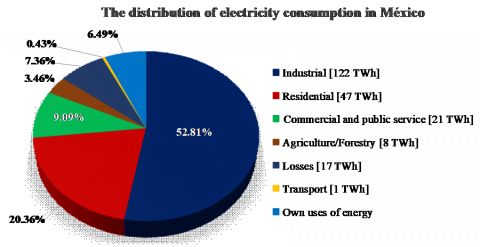Figure 1. Distribution of electricity consumption in Mexico in 2017

The wide use of induction motors is due to their simple and robust construction, lower cost and because they provide excellent service with little maintenance. However, its main drawback is its limitation to regulate its speed. An alternative solution is to use direct current motors which will result in a more expensive solution, due to their high maintenance costs .

## 2.pngFigure 2. Distribution of electricity consumption in the industrial sector

Fortunately, the use of power inverters has made it possible to control the speed of these induction motors to adapt them to the demand of the load. Even small changes in the motor speed result in significant changes in power consumption [4, 5].

The inverters generate an alternating current from a DC power source. Ideally, an inverter should provide an output voltage with a pure sine waveform, at the desired frequency and magnitude. However, it is possible that it can contain harmonic contamination, causing the motors to operate at a reduced efficiency and performance .

Power semiconductor devices such as switches (e.g. BJT, MOSFET, IGBT, etc.) are used in the construction of the inverter. By applying a trigger signal to the gate, these devices operate in the cut-off and saturation regions. The inverter output voltage signal is obtained by varying the conduction time of these switching devices. Using a suitable switching sequence in the inverter contributes to reduce Total Harmonic Distortion (THD) in the output voltage delivered to the load.

In this paper, four Pulse Width Modulation (PWM) techniques applied to a dual inverter are compared by simulation in order to analyze their advantages and limitations. The rest of the paper is organized as follows: section 2 contains a brief description of the advantages and disadvantages of the different inverter topologies used to vary the speed of induction motors. Section 3 describes the operation of the open-end winding induction motor. Section 4 includes a brief analysis of the operation of the dual inverter. The PWM techniques used in this paper are explained in Section 5. The simulation results obtained, and a comparative analysis of the output voltage waveform obtained by the dual inverter are presented in Section 6. Finally, the conclusions are presented in Section 7.

2. Power Inverters

Figure 3 shows a conventional three-phase inverter, composed of three branches of power semiconductor devices (PSDs). Depending on the switching sequence used in the PSDs, the inverter is capable of producing three levels in the output voltage waveform. Therefore, the thermal and electrical stresses in semiconductor devices are high .

The induction motors supplied by the inverter present various problems, such as noise, vibration, motor bearing failure, overvoltage, among others. As the switching frequency of semiconductor devices increases, these drawbacks increase .

## 3.png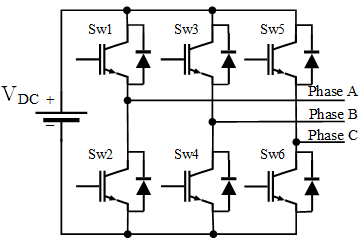Figure 3. Three-phase full bridge inverter (Conventional)

An alternative to overcome the problems associated with conventional inverters is to use multilevel topologies. Figure 4 shows a three-phase cascade multilevel inverter. Multilevel inverters synthesize the output voltage in a stepped waveform. The main advantages offered by these topologies are lower switching losses, less stress on semiconductor devices, a considerable increase in power capacity, higher output signal quality and THD reduction, among others . However, its main disadvantage is the number of power semiconductor devices used.

In the literature, three classic multilevel inverter topologies have been proposed: Neutral Point Clamped Multilevel Inverter (NPC-MLI), Flying Capacitor Multilevel Inverter (FC-MLI), Cascaded H-bridge Multilevel Inverter (CHB-MLI). A disadvantage of these inverters is that as the number of levels increases, the number of semiconductor devices also increases significantly, and the modulation techniques required are more complex [8, 9]. However, using the topology of the dual inverter is possible to obtain the same number of levels as in a multilevel inverter, but using a smaller number of power semiconductor devices (PSDs). For example, a three-phase five-level CHB-MLI uses 24 PSDs. In contrast, the five-level dual inverter uses only 12 PSDs.

## 4.png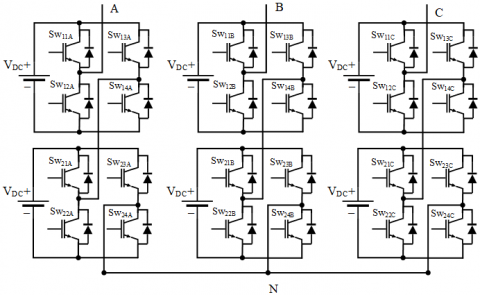Figure 4. Three-phase 5-level cascade H-bridge multilevel inverter

3. Open-End Winding Induction Motor

Currently, the three-phase induction motor in a star-connection (Y-connection) is widely used in the industry (See Figure 5-I). The Y-connection is achieved by joining one terminal of each one of the three coils (A', B', C' of Figure 5-II) to a common point, which we call a neutral point (N). This point is usually connected to the earth ground.

In the Y-connection, each phase behaves as a single-phase system and consumes 4 to 8 times the nominal current when starting-up since it requires a very large initial power to overcome the motor statics. As the motor rotates, the current decreases until it reaches the nominal current. One way to reduce the starting current (excessive power consumption) is to use a low-voltage start-up circuit. An interesting proposal for reducing the voltage at the motor terminals is to use open-end windings.

## 5.png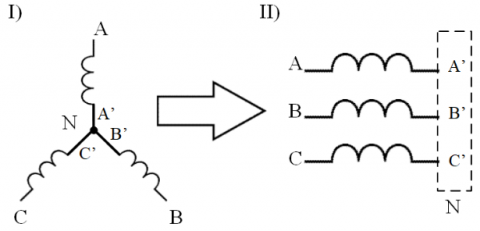Figure 5. Connection diagram: I) Star (Y) and II) Open-end windings

Open-end windings are obtained by disconnecting the neutral point of the conventional Y-connection motor, as shown in Figure 5-II. This configuration has six terminals instead of three and, requires two conventional three-phase full bridge inverters to powered both sides of the induction motor.

4. Dual Inverter

In the last decade, the dual inverter has gained much importance as a speed variation system in induction motors. The dual inverter consists of two conventional three-phase inverters (anti-parallel mounted), each powered by a DC power supply, as shown in Figure 6. The separate DC power supply is used for individual inverters to block the flow of harmonic currents.

The main benefits offered by this topology are: precise voltage control at the motor terminals [10-12], improved output voltage (with stepped multilevel waveforms) , and improved motor performance in terms of speed range [14, 15].

In addition, the dual topology can include a fault reconfiguration system, increasing its reliability. This system will allow the motor to continue to operate in conjunction with the non-faulted inverter, in the event of a fault in any of the inverters (i.e. double material redundancy).

In a dual inverter, there are 12 power semiconductor devices, and each power switch has two possible switching states: "ON" and "OFF". Therefore, there are 2^12=4096 possible combinations or switching states. Of these possible states, there are many states that are not allowed because they are short or open circuit states.

## 6.png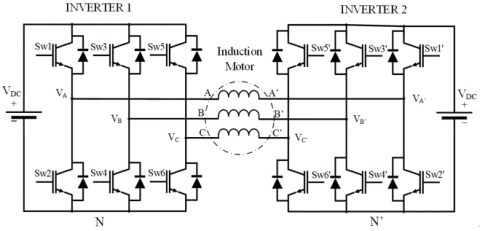Figure 6. Dual inverter feeding an open-end winding induction motor

Table 1. Switching states of the three-phase inverter

 State Switching Function S0 0 0 0 S1 1 0 0 S2 1 1 0 S3 0 0 0 S4 0 1 1 S5 0 0 1 S6 1 0 1 S7 1 1 1

In a conventional three-phase inverter, there are eight possible switching states (see Table 1), where the states S1-S6 are called active switching states, which connect the load to a potential difference (VDC+, VDC-). The remaining switching states S0 and S7, are called zero states when these are activated, they short-circuit the load to a zero potential difference using the lower or upper switches . The configuration of the eight possible operating states of the conventional three-phase inverter is shown in Figure 7.

A conventional three-phase inverter has 8 possible switching states and the dual inverter (see Figure 7) consists of two conventional inverters connected to a load. Therefore, it is possible to obtain 64 possible switching states. Three voltage levels are obtained at any phase of the load, i.e. the VAA' voltage can reach the -VDC, 0, +VDC voltage levels. The phase voltages of inverter 1 are VAN, VBN and VCN. For inverter 2, the phase voltages are: VA’N’, VB’N’, VC’N’.

The line voltage (phase-to-phase voltage) is measured across two windings. Therefore, there is a potential difference and is expressed as follows:

${{\text{V}}_{\text{AA }\!\!'\!\!\text{ }}}\text{=}{{\text{V}}_{\text{AN}}}\text{-}{{\text{V}}_{\text{A }\!\!'\!\!\text{ N }\!\!'\!\!\text{ }}}$     (1)

${{\text{V}}_{\text{BB }\!\!'\!\!\text{ }}}\text{=}{{\text{V}}_{\text{BN}}}\text{-}{{\text{V}}_{\text{B }\!\!'\!\!\text{ N }\!\!'\!\!\text{ }}}$     (2)

${{\text{V}}_{\text{CC }\!\!'\!\!\text{ }}}\text{=}{{\text{V}}_{\text{CN}}}\text{-}{{\text{V}}_{\text{C }\!\!'\!\!\text{ N }\!\!'\!\!\text{ }}}$     (3)

In a conventional three-phase inverter, there are eight possible switching states (see Table 1), where the states S1-S6 are called active switching states, which connect the load to a potential difference (VDC+, VDC-). The remaining switching states S0 and S7, are called zero states when these are activated, they short-circuit the load to a zero potential difference using the lower or upper switches . The configuration of the eight possible operating states of the conventional three-phase inverter is shown in Figure 7.

## 7.png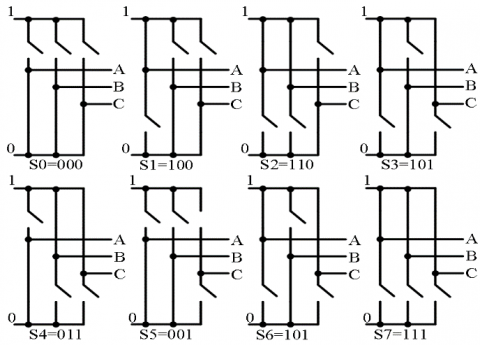Figure 7. Configuration of the eight possible operating states of the conventional three-phase inverter

## 8.png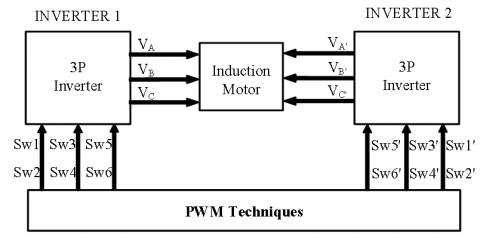Figure 8. Dual inverter configuration scheme using PWM techniques

The ideal waveform of the output voltage at the inverter output is a pure sine waveform. However, has harmonic distortion that can be reduced by using a switching sequence suitable for power semiconductor devices. An alternative to reduce harmonic distortion in high-power converters is to use PWM techniques as shown in Figure 8.

5. PWM Techniques

There are a variety of modulation techniques used in the control of three-phase inverters; one of the most widely used is Pulse Width Modulation (PWM). The PWM technique consists of modifying the duty cycle of a periodic signal (e.g. a sinusoidal waveform), to generate a square wave of a constant frequency with variable pulse duration, controlling the amount of energy delivered to the load. The output voltage changes as a function of the pulse width of the duty cycle, which can vary from 0 to 100% of the wave period.

Using PWM techniques is possible to reduce the switching losses, improving the efficiency of the converter and reducing the harmonic content in the output voltage waveform. In electric drives, the harmonic content generates undesirable effects (e.g. heating in the motor windings, and additional losses due to negative sequence effects.), deteriorating the useful lifetime of the motor.

To commutate the PSDs of each three-phase inverter in the dual topology, three sinusoidal modulation signals (one per phase) are required, shifted 120° with respect to each other. Furthermore, depending on the number of levels desired at the output, two or more carrier signals are required. Figure 9 shows the PWM modulation scheme for a three-phase 2-level inverter.

## 9.png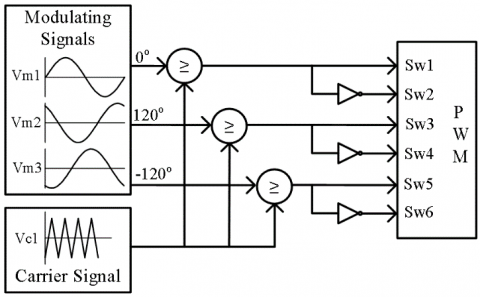Figure 9. PWM modulation scheme for a three-phase 2-level inverter (conventional)

In this paper, a comparative analysis of four variants of PWM techniques, widely used as drives in induction motors, was carried out. The PWM techniques analyzed are briefly described below:

5.1 Sinusoidal pulse width modulation (SPWM) technique

In the Sinusoidal Pulse Width Modulation (SPWM) technique, modulation is achieved by comparing a sinusoidal signal with a triangular carrier wave to generate trigger signals for semiconductor devices.

The modulating signal (Vm1) is given by:

$\mathrm{V}_{\mathrm{m}_{1}}=\mathrm{A}_{\mathrm{m}_{1}} \sin \left(2 \pi \mathrm{f}_{\mathrm{m}_{1}} \mathrm{t}\right)$     (4)

where,

Am1: Amplitude of the modulating sinusoidal signal (Volts).

fm1: Frequency of the modulating sinusoidal signal (Hertz, Hz).

t: Time (seconds, s).

The carrier signal (Vc1) is given by:

$\mathrm{V}_{\mathrm{cl}}=\mathrm{A}_{\mathrm{c}}\left[\frac{2}{\pi} \arcsin \left(\sin \left(2 \pi \mathrm{f}_{\mathrm{c}} \mathrm{t}-\frac{\pi}{2}\right)\right)\right]$     (5)

where,

Ac: Amplitude of the triangular carrier signal (Volts).

fc: Frequency of the triangular carrier signal (Hertz, Hz).

The amplitude modulation (m) ratio between the modulating signal and the carrier is given by:

$\mathrm{m}=\mathrm{A}_{\mathrm{m}_{1}} / \mathrm{A}_{\mathrm{c}}$     (6)

The frequency modulation (fm) ratio can be expressed as follows:

$\mathrm{f}_{\mathrm{m}}=\mathrm{f}_{\mathrm{m}_{1}} / \mathrm{f}_{\mathrm{c}}$     (7)

Figure 10 shows the signals for the phase A, using the SPWM technique with m=1 and number of pulses fm=50.

## 10.png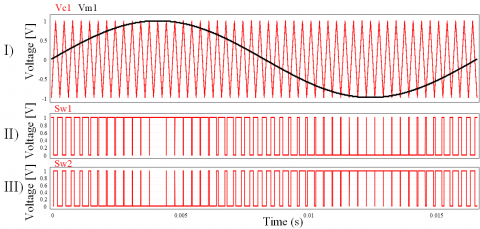Figure 10. Signals using SPWM. I) Modulating and carrier signals for phase A, II) and III) Switching signals for Sw1 and Sw2, respectively

5.2 Alternative phase opposition disposition PWM (APOD-PWM) technique

In the APOD-PWM technique, the carrier signal is replaced by two carrier signals shifted one from the other by 180°. In addition, the carrier signals are offset: one on a positive DC level and the other on a negative DC level.

The modulating signal (Vm1) is expressed in the same way as the previous technique in Equation (4).

The carrier signals (Vc1 and Vc2) are given by:

$\mathrm{V}_{\mathrm{c}_{1}}=\mathrm{A}_{\mathrm{c}_{1}}\left[\frac{2}{\pi} \arcsin \left(\sin \left(2 \pi \mathrm{f}_{\mathrm{c}} \mathrm{t}-\frac{\pi}{2}\right)\right)\right]+\mathrm{A}_{\mathrm{c}_{1}}$     (8)

$\mathrm{V}_{\mathrm{c}_{2}}=\mathrm{A}_{\mathrm{c}_{2}}\left[\frac{2}{\pi} \arcsin \left(\sin \left(2 \pi \mathrm{f}_{\mathrm{c}} \mathrm{t}+\frac{\pi}{2}\right)\right)\right]-\mathrm{A}_{\mathrm{c}_{2}}$     (9)

where,

Ac1: Amplitude of the triangular carrier signal number one (Volts).

Ac2: Amplitude of the triangular carrier signal number two (Volts).

fc: Frequency of the triangular carrier signal (Hertz, Hz).

t: Time (seconds, s).

As a rule, the magnitude value of the amplitude of the carrier signals must be equal, therefore Vc1 = Vc2 =Vc.

The amplitude modulation (m) ratio between the modulating signal and the carrier is given by:

$\mathrm{m}=\mathrm{A}_{\mathrm{m}_{1}} /\left(2 \mathrm{A}_{\mathrm{c}}\right)$     (10)

The frequency modulation (fm) ratio can be expressed by Eq. (7).

Figure 11 shows the signals for the phase A, using the APOD-PWM technique with m=1 and fm=50.

## 11.png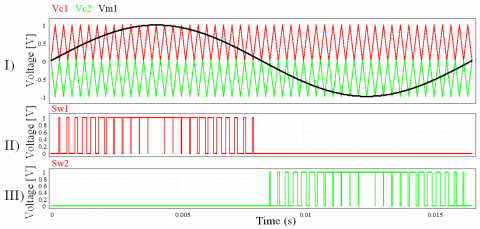Figure 11. Signals using APOD-PWM. I) Modulating and carrier signals for phase A, II) and III) Switching signals for Sw1 and Sw2, respectively

5.3 Third harmonic injection PWM (THI-PWM) technique

In the THI-PWM technique, the modulating signal is a composite sine wave signal, resulting from the sum of two sine wave signals. For the composite sine wave, it is integrated by a fundamental signal, and a zero-sequence signal with amplitude 1⁄6 and with three times the frequency of the fundamental signal.

The modulating signal (Vm1) is given by:

$\mathrm{V}_{\mathrm{m}_{1}}=\mathrm{A}_{\mathrm{m}_{1}} \sin \left(2 \pi \mathrm{f}_{\mathrm{m}_{1}} \mathrm{t}\right)-\frac{\mathrm{A}_{\mathrm{m}_{1}}}{6} \sin \left(2 \pi \mathrm{f}_{\mathrm{m}_{1}} \mathrm{t}\right)$     (11)

The amplitude modulation (m) ratio between the modulating signal and the carrier is given by Eq. (10).The carrier signals (Vc1 and Vc2) are given by the Eqns. (8) and (9) respectively.

The frequency modulation (fm) ratio can be expressed by Eq. (7).

Figure 12 shows the signals for the phase A, using the THI-PWM technique with m=1 and fm=50.

## 12.png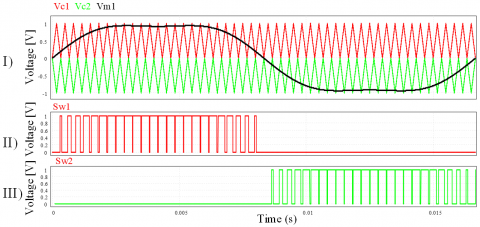Figure 12. Signals using THI-PWM. I) Modulating and carrier signals for phase A, II) and III) Switching signals for Sw1 and Sw2, respectively

5.4 Carrier based space vector PWM (CBSV-PWM) technique

In this technique, the modulating signal results from the sum of a sinusoidal signal at a fundamental frequency with a triangular signal at three times frequency and 1/4 the amplitude of the sinusoidal signal, and compared to a periodic triangular carrier signal.

The modulating signal (Vm1) is given by:

$\mathrm{v}_{\mathrm{x}}=\mathrm{A}_{\mathrm{m}_{1}} \sin \left(2 \pi \mathrm{f}_{\mathrm{m}_{1}} \mathrm{t}\right)$     (12)

$\mathrm{V}_{\mathrm{m}_{1}}=\mathrm{v}_{\mathrm{x}}-\frac{1}{2}\left[\max \left(\mathrm{v}_{\mathrm{x}}\right)+\min \left(\mathrm{v}_{\mathrm{x}}\right)\right]$     (13)

The carrier signals (Vc1 and Vc2) are given by the Eqns. (8) and (9) respectively.

The amplitude modulation (m) ratio between the modulating signal and the carrier is given by Eq. (10).

The frequency modulation (fm) ratio can be expressed by Eq. (7).

Figure 13 shows the signals for the phase A, using the CBSV-PWM technique with m=1 and fm=50.

## 13.png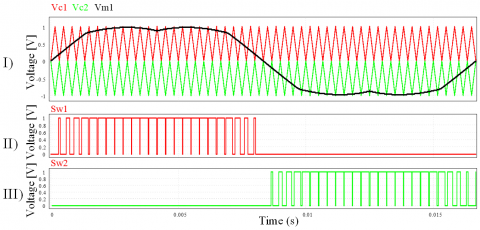Figure 13. Signals using CBSV-PWM. I) Modulating and carrier signals for phase A, II) and III) Switching signals for Sw1 and Sw2, respectively

6. Simulation Results

In this paper, a comparative analysis of the output voltage behaviour of a three-phase dual inverter was performed. The four modulation techniques (SPWM, APOD-PWM, THI-PWM, CB-SVPWM), briefly described in Section 5, were applied. The comparative analysis was carried out using the simulation results obtained with the PSim software. The schematic diagram is shown in Figure 14 and the simulation parameters used are shown in Table 2.

Figure 14 shows two H-bridge circuits connected to a load of a three-phase induction motor. Each circuit is labelled with its respective name "Inverter 1" and "Inverter 2", each traditional three-phase inverter circuit is composed of six MOSFETs devices. For the simulation of this circuit, the SPWM technique (briefly described in Section 5.1) was initially applied to both inverters. However, for Inverter 2, phase shift angles (α) varying from 0° to 180° were added. The triangular shapes shown in the schematic simulation diagram, placed between the comparators and the terminals of the MOSFETs gates, are called on/off controllers. Additionally, because the gate signals from devices in the same branch must be complementary, an inverter type digital gate or NOT is placed.

Table 2. Switching states of the three-phase inverter

 Parameter Value Input voltage (VDC) 310 V Load resistance 100 Ω Load inductance 0.01 mH Switching frequency 3 kHz Output voltage frequency 60 Hz Simulation time 50ms Simulation step 50us Phase shift angle (α) From 0° to 180°

## 14.png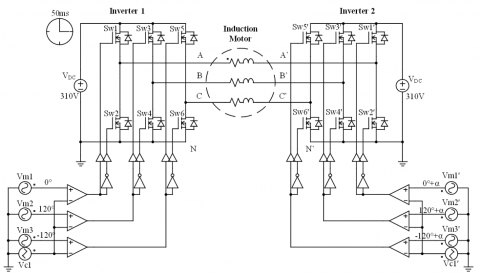Figure 14. Schematic diagram for the simulation of the dual inverter feeding an open-end winding induction motor

6.1 Simulation results in stable operating mode

Figure 15 shows the voltage obtained using the SPWM technique, measuring at the motor terminals (VAA’) when inverter 2 is with a 180° phase shift (Figure 15-I) and when it is at 90° (Figure 15-II), with respect to inverter 1. As can be seen, when varying the phase angle between the inverters, the voltage at the load (the voltage provided by both inverters) is affected proportionally. When the phase shift angle of inverter 2 is 180° (see Figure 15-I), it can be seen that the modulation waveform covers almost the entire period. In contrast, when the phase shift angle of inverter 2 is 90° (see Figure 15-II), the voltage modulation waveform covers about half of the period. Finally, when the phase shift angle is 0° on inverter 2, the voltage modulation waveform will appear at the 0V level.

Figure 16 shows the waveform of the VAB voltage obtained using the CB-SVPWM technique, a 5-level voltage waveform is observed. It is important to note, that each three-phase inverter provides a 3-level waveform (a positive level, a negative level, and the zero level). The figure shows only 5 levels instead of 6 because the zero levels are repeated in both inverters.

## 15.png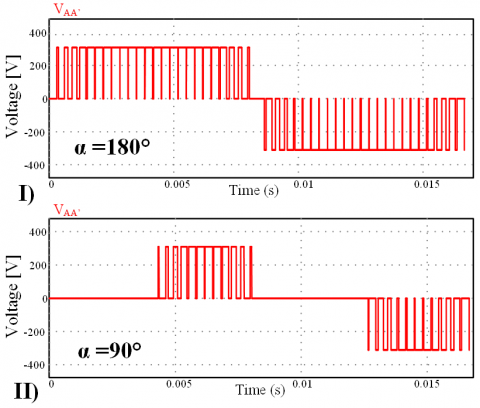Figure 15. Voltage obtained using the SPWM at the motor terminals (VAA’). I) 180° phase shift and, II) 90° phase shift

## 16.png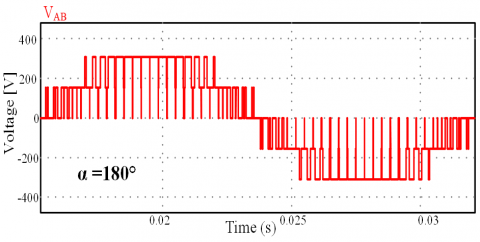Figure 16. Voltage VAB obtained using the CB-SVPWM technique

6.2 Comparative Results

To verify the behaviour of the dual inverter output voltage (VAA’), a comparative analysis was performed using four modulation techniques. These techniques were briefly described in Section 5. In order to carry out the comparison properly, the following conditions were maintained for each inverter:

Inverter 1.-The modulation technique used remained fixed, i.e. Phase A with an angle of 0° and Phases B and C at -120° and 120° respectively, taking Phase A as a reference.

Inverter 2.- The same modulation technique used in Inverter 1 was used with a phase shift (α) from 0° to 180° with respect to Phases A, B and C of inverter 1, increasing in 10° intervals.

Figure 17 shows the behaviour of the output voltage waveform VAA’, varying the phase shift angle of inverter 2, using the four modulation techniques briefly described in Section 5. The comparative results obtained from the simulation. As can be observed, the SPWM technique is best suited for the DC bus because it gets a higher output voltage value. However, exhibits a non-linear behaviour, which complicates the implementation of some traditional control technique.

The APOD-PWM technique shows a behaviour with greater linearity compared to the other techniques analysed. However, it also has the lowest voltage levels. The THI-PWM and CB-SVPWM techniques exhibit relatively linear and balanced behaviour with respect to each other. However, they have higher voltage values compared to those obtained with APOD-PWM modulation.

## 17.png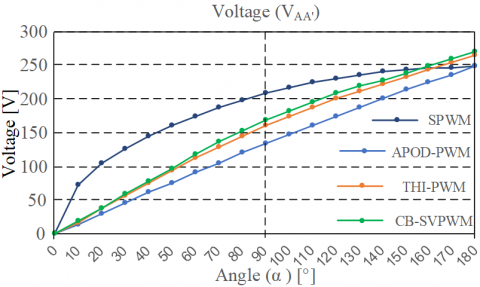Figure 17. Behaviour of the voltage VAA’, varying the phase shift angle of inverter 2, using four modulation techniques

7. Conclusions

In this paper, a comparative analysis of the output voltage behaviour of a three-phase dual inverter was performed, using an open-end winding induction motor as load. Four modulation techniques (SPWM, APOD-PWM, THI-PWM, CB-SVPWM) were applied. The comparative analysis was carried out using the simulation results obtained with the PSim software.

According to the results obtained, with the SPWM technique a more effective use of the DC bus is obtained during a wider modulation range (except at an angle of 180°), exhibiting non-linear behaviour which complicates the control strategy. In contrast, the THI-PWM and CB-SVPWM techniques provide a higher voltage at an angle of 180° and exhibit linear behaviour.

The APOD-PWM technique exhibited linear behaviour. However, exhibited lower voltage levels compared to the other techniques.

From the comparative analysis carried out, it can be concluded that the CB-SVPWM technique obtained a better trade-off between the complexity of the control and the output voltage.

References

 Hernández, F.M., Vera, M.S., De la Vega Navarro, Á. (2016). La reforma energética de 2013/2014 y el desarrollo industrial en México: contenidos, implicaciones y propuestas. Análisis Económico, 31(78): 7-32.

 Gutiérrez, L.D.J.R., Fragoso, M.M. (2012). La generación de energía eléctrica en México. Tecnología y Ciencias del Agua, 3(4): 197.

 Cruz, P.P., López, J.S. (2008). Máquinas Eléctricas: y Técnicas Modernas de Control (1a. Edición ed.). México: Alfaomega.

 Liu, Z.J., Wu, W. (2017). A novel control method for five-level H-bridge/neutral point clamped inverter. European Journal of Electrical Engineering, 19(3-4): 235-245. https://doi.org/10.3166/EJEE.19.235-245

 Yahiaoui, A., Iffouzar, K., Himour, K., Ghedamsi, K. (2019). Comparison of different multilevel voltage source inverter topologies on induction motor energy quality. European Journal of Electrical Engineering, 21(4): 367-372. https://doi.org/10.18280/ejee.210404

 Wang, L., Hao, S.H., Hao, M.H., Song, B.Y. (2016). A harmonic injection SPWM method for the high-responsive PMSM control system. International Journal of Electronics, 103(1): 130-146. https://doi.org/10.1080/00207217.2015.1036312

 García, J.A.E. (2009). Modulador PWM en FPGA para un inversor multinivel en cascada. Thesis, Departamento de Ingeniería Electrónica. Centro Nacional de Investigación y Desarrollo Tecnológico.

 Freddy, C.P., Hugo, C.G., Víctor, S.H., Roberto, A.O., Emmanuel, T.M. (2009). Tendencias actuales en el diseño de convertidores de potencia para aplicaciones en fuentes de energía renovables. Ingeniería Eléctrica, Electrónica y Computación, 7(2): 1-7.

 Nirsha, K.I., Rajeevan, P.P. (2017). A direct torque control scheme for dual inverter fed induction motor drive with a common DC voltage source. IECON 2017-43rd Annual Conference of the IEEE Industrial Electronics Society, pp. 1674-1679. https://doi.org/10.1109/IECON.2017.8216284

 Smith, I., Haque, R.U., Tavakoli, A., Salmon, J. (2017). Power factor control for high efficiency operation of an open-ended winding motor using a dual inverter drive with a floating bridge. 2017 IEEE Applied Power Electronics Conference and Exposition (APEC), Tampa, FL, USA, pp. 1935-1941. https://doi.org/10.1109/APEC.2017.7930962

 Edpuganti, A., Rathore, A.K. (2015). New optimal pulse width modulation for single DC-link dual-inverter fed open-end stator winding induction motor drive. IEEE Transactions on Power Electronics, 30(8): 4386-4393. https://doi.org/10.1109/TPEL.2014.2353415

 Chowdhury, S., Wheeler, P., Gerada, C., Arevalo, S.L. (2015). A dual inverter for an open end winding induction motor drive without an isolation transformer. 2015 IEEE Applied Power Electronics Conference and Exposition (APEC), Charlotte, NC, USA, pp. 283-289. https://doi.org/10.1109/APEC.2015.7104364

 Kumar, G.N., Srinivas, S. (2018). Carrier based PWM methods for CMV elimination in open-end winding induction motor drive. 2018 IEEE 12th International Conference on Power Electronics and Power Engineering (CPE-POWERENG), Doha, Qatar, pp. 1-6. https://doi.org/10.1109/CPE.2018.8372599

 Rao, R.S., Chaitanya, B.N., Saichand, N., Somasekhar, V.T. (2014). Comparative evaluation of SVPWM strategies for a dual inverter fed open-end winding induction motor drive with a single DC power supply. IECON 2014-40th Annual Conference of the IEEE Industrial Electronics Society, Dallas, TX, USA, pp. 443-449. https://doi.org/10.1109/IECON.2014.7048538

 Le, J., Li, X.R., Wang, C., Zhang, H., Gao, C. (2018). Novel compound PWM strategy of a four-leg shunt active power filter. International Journal of Electronics, 105(12): 1993-2008. https://doi.org/10.1080/00207217.2018.1494328

 Loutfi, B. (2019). Faults detection and diagnosis of multilevel inverter based on signal processing. Traitement du Signal, 6(1): 37-44. https://doi.org/10.18280/ts.360105